# 7 1 Ratio and Proportions Ratio and Proportion

• Slides: 41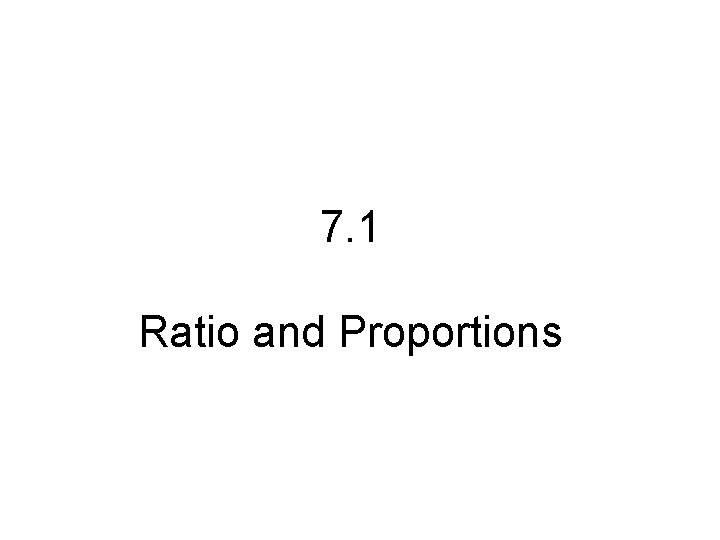7. 1 Ratio and ProportionsRatio and Proportion • ________________________________________ • ____________________ Word form: Colon form: Fraction form: ____________________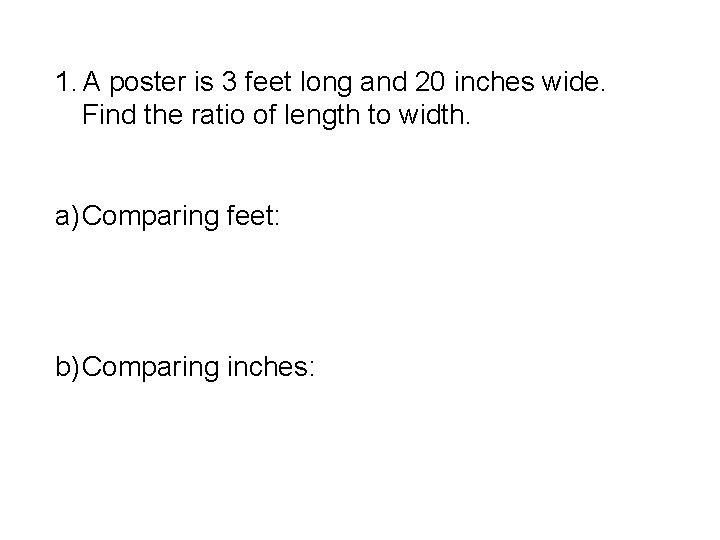1. A poster is 3 feet long and 20 inches wide. Find the ratio of length to width. a) Comparing feet: b) Comparing inches: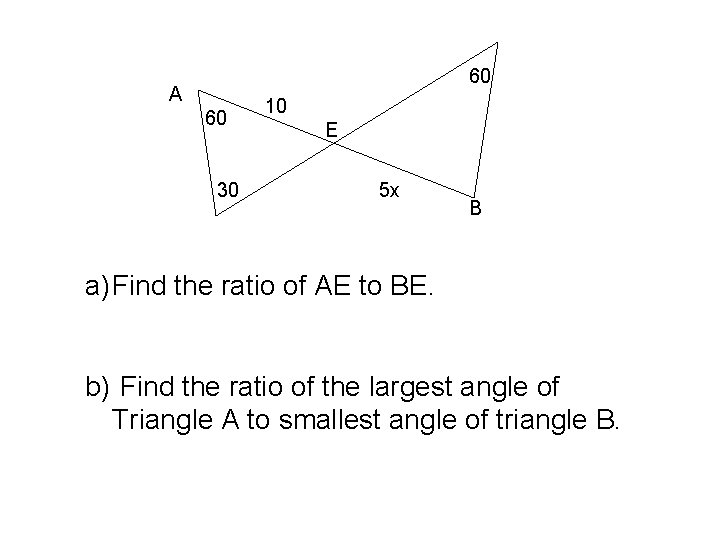60 A 60 30 10 E 5 x B a) Find the ratio of AE to BE. b) Find the ratio of the largest angle of Triangle A to smallest angle of triangle B.3. A telephone pole 7 meters tall snaps into two parts. The ratio of the two parts is 3: 2. Find the length of each part.A B 6 D 10 CThe measures of the angles of a triangle are in the ratio of 3: 4: 5. Find the measures of each angle.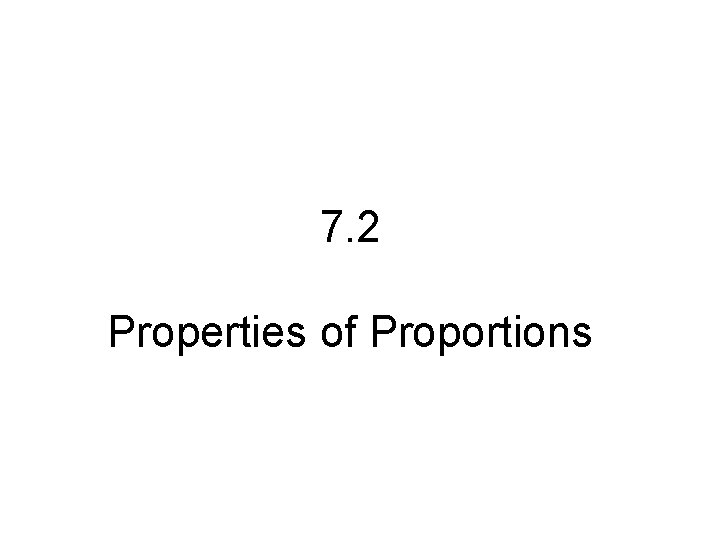7. 2 Properties of Proportions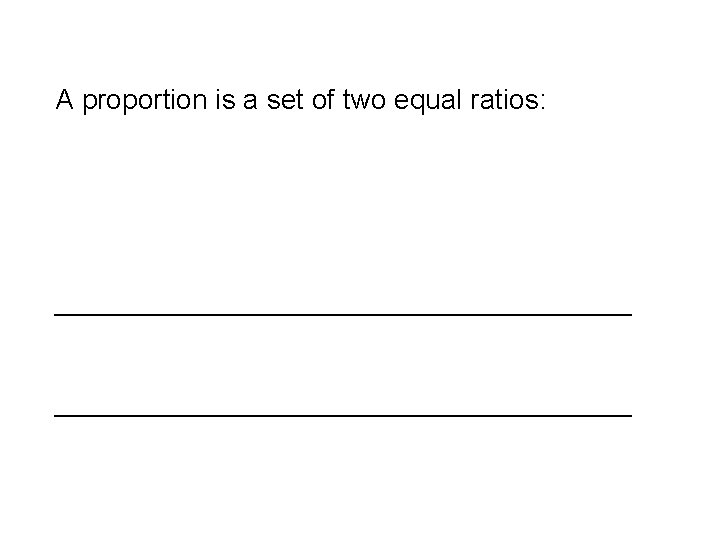A proportion is a set of two equal ratios: _____________________________________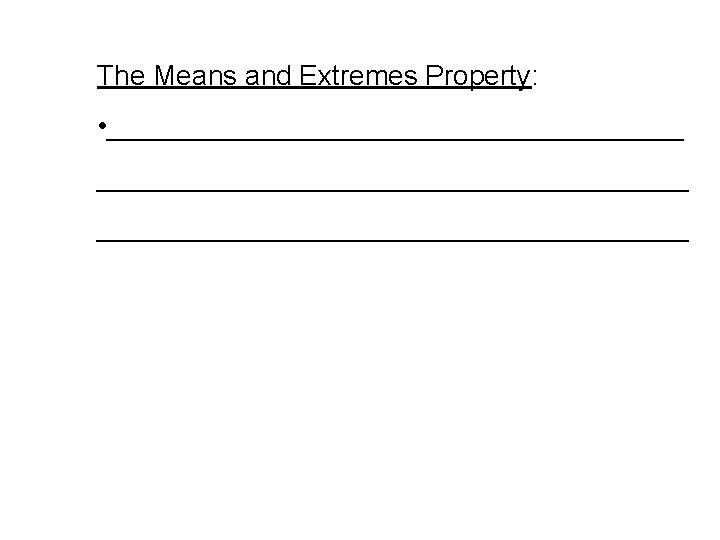The Means and Extremes Property: • ______________________________________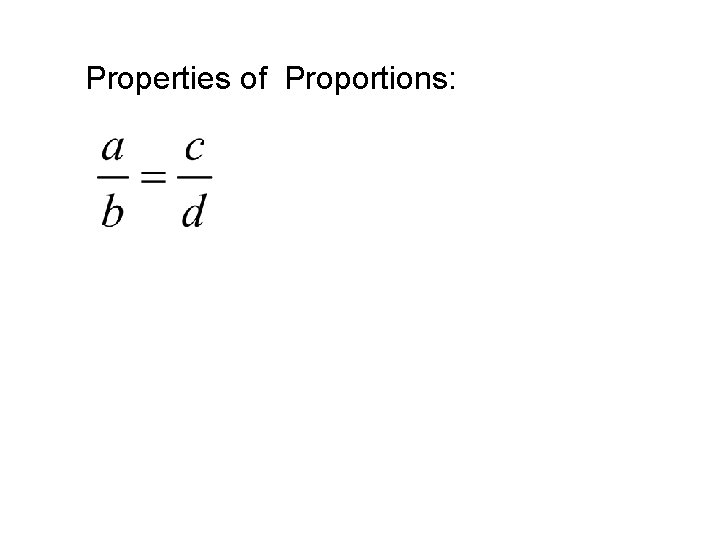Properties of Proportions: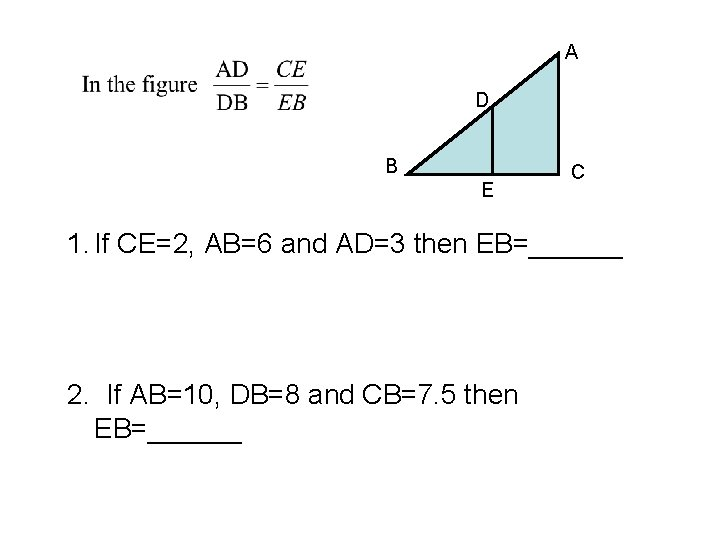A D B E C 1. If CE=2, AB=6 and AD=3 then EB=______ 2. If AB=10, DB=8 and CB=7. 5 then EB=______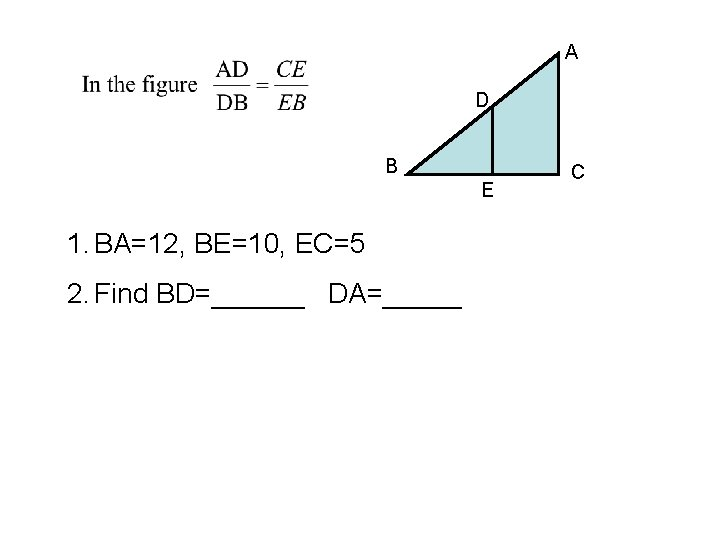A D B E 1. BA=12, BE=10, EC=5 2. Find BD=______ DA=_____ C7. 3 Similar Polygons__________________________________________ Two polygons are similar if their vertices can be paired so that: • __________________________________________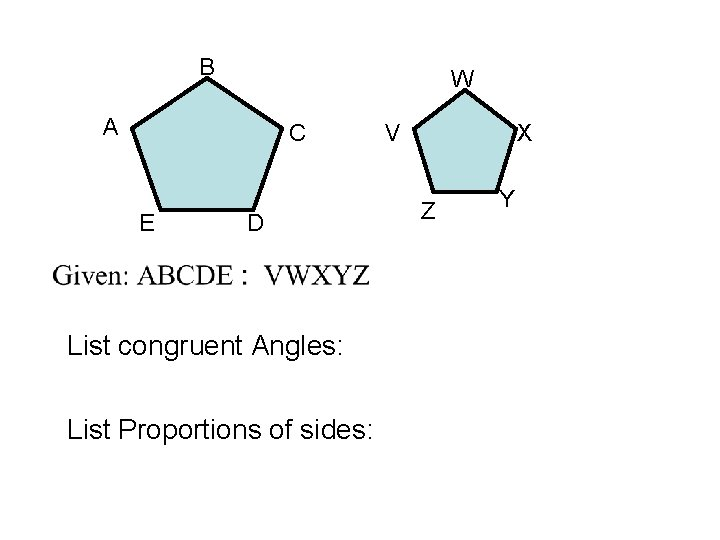B W A C E D List congruent Angles: List Proportions of sides: V X Z YIf polygons are similar then the ratio of the lengths of two corresponding sides is called the Scale Factor 12 D C x z A 30 B a) Scale Factor: D` 30 y C` 22 A` 50 B` a) Find x, y, z: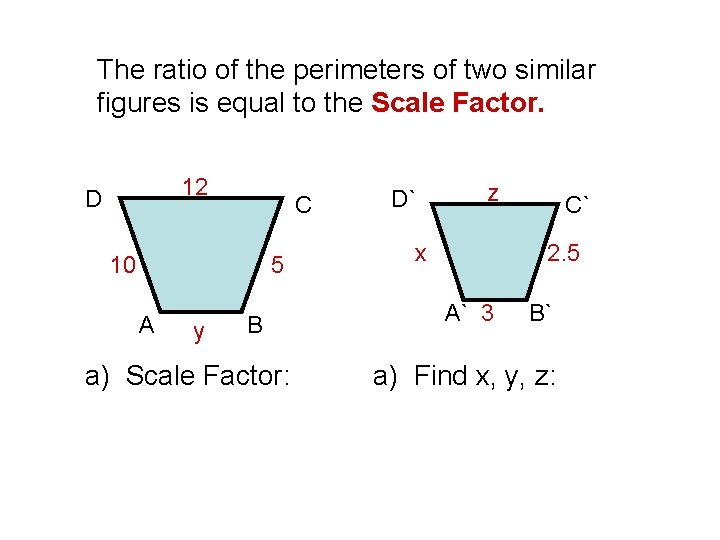The ratio of the perimeters of two similar figures is equal to the Scale Factor. 12 D C 10 5 A y B a) Scale Factor: D` z x C` 2. 5 A` 3 B` a) Find x, y, z: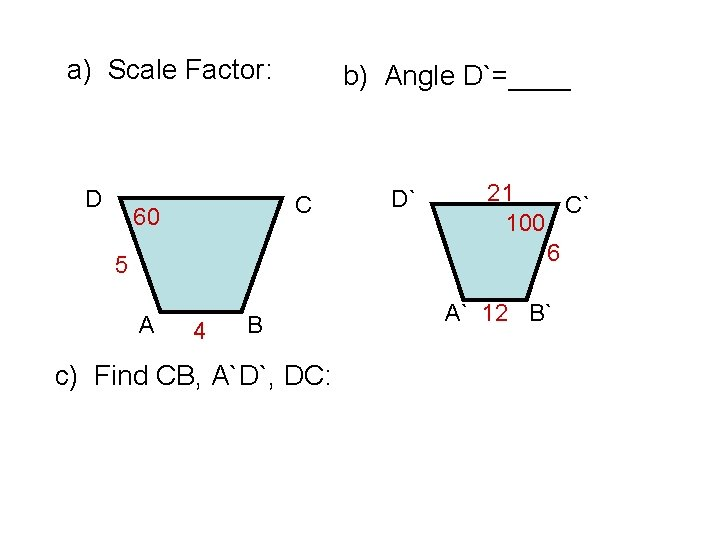a) Scale Factor: D b) Angle D`=____ C 60 5 A 4 B c) Find CB, A`D`, DC: D` 21 C` 100 6 A` 12 B`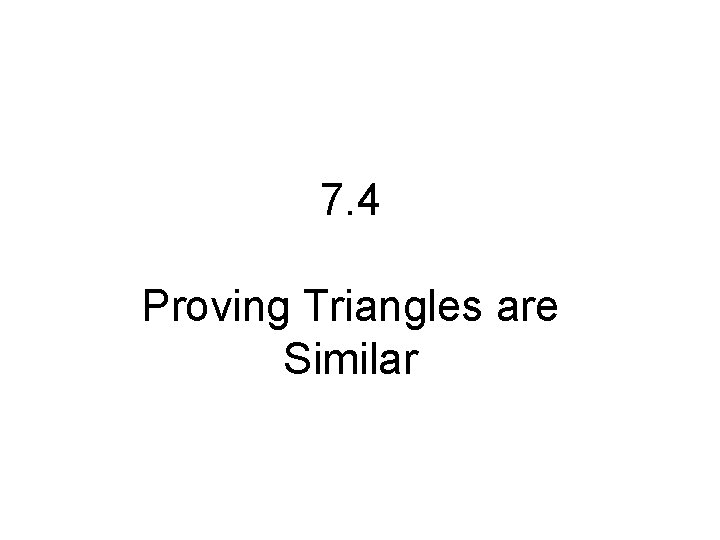7. 4 Proving Triangles are Similar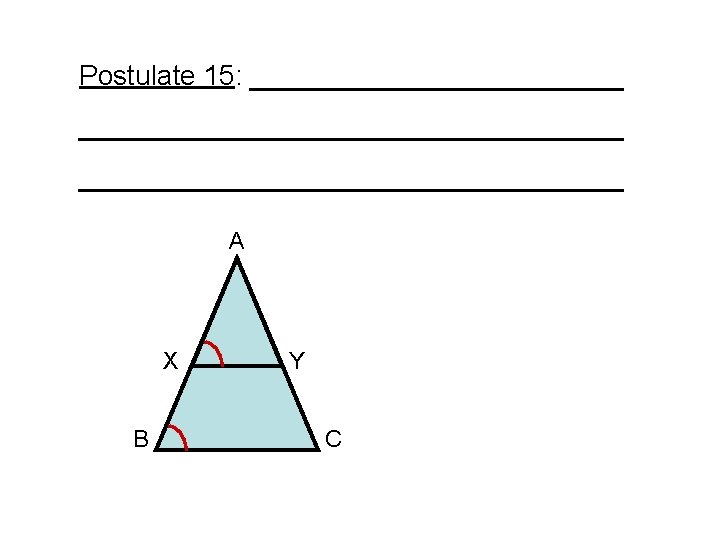When there are triangles within Trianlges: • _______________________________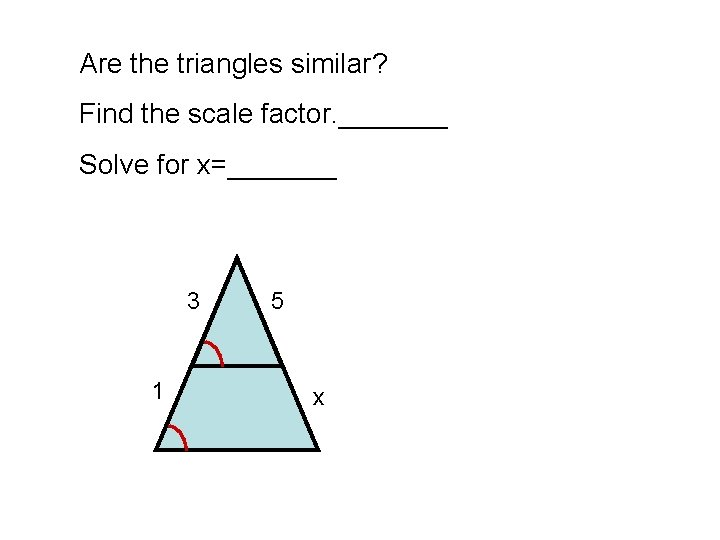Are the triangles similar? Find the scale factor. _______ Solve for x=_______ 3 1 5 xFind x=______ and y=______: Scale Factor=____ x 8 3 y 3 4A D O C B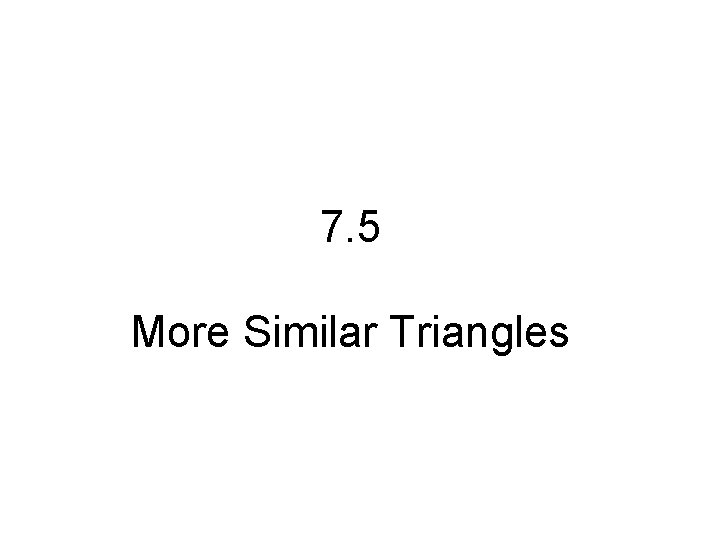7. 5 More Similar Triangles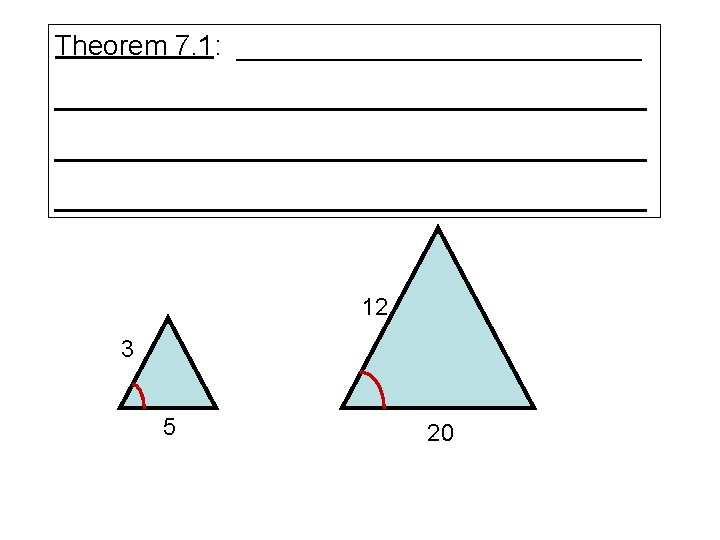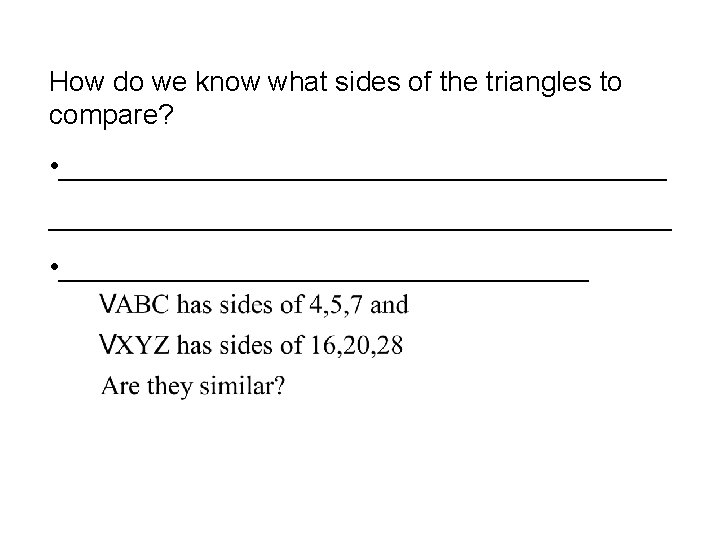How do we know what sides of the triangles to compare? • ________________________________________ • _________________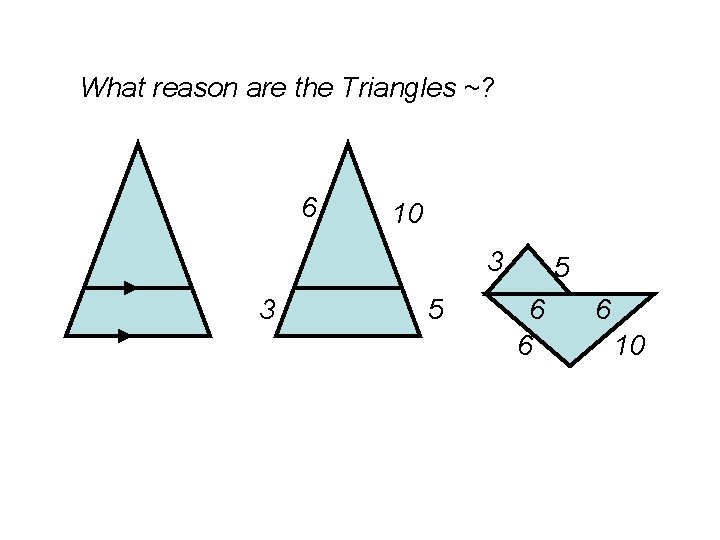What reason are the Triangles ~? 6 10 3 3 5 5 6 6 6 10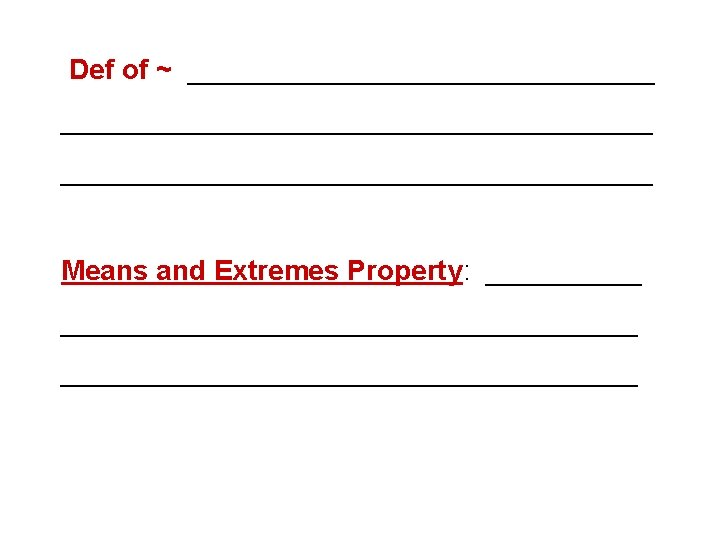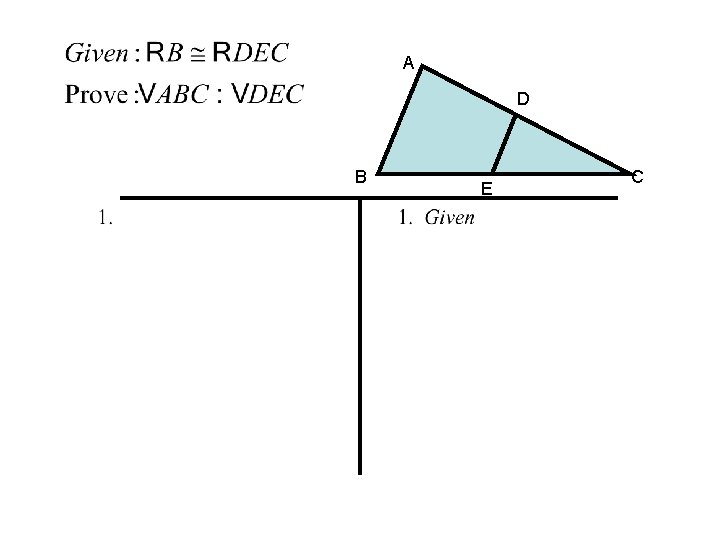A D B E C10 9 x y 15 16 Solve for x and y: Scale Factor? ____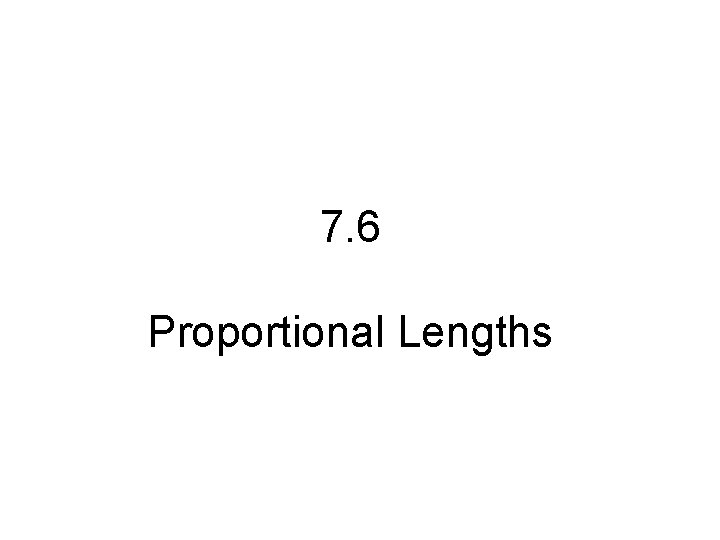7. 6 Proportional Lengths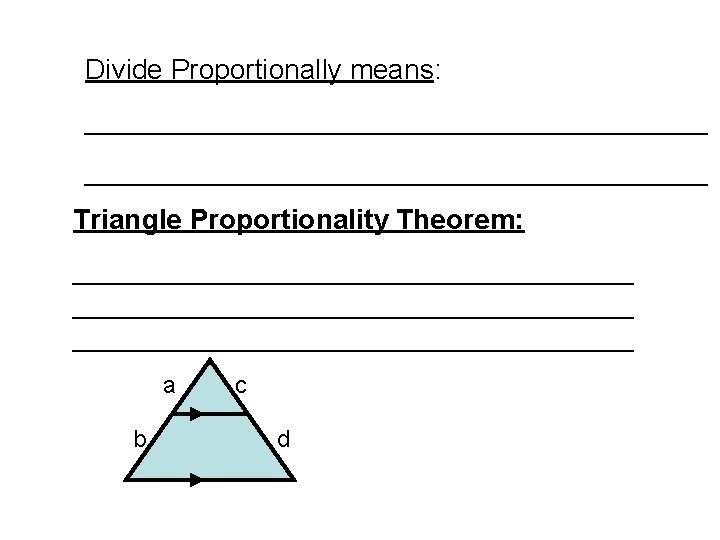Divide Proportionally means: ________________________________________ Triangle Proportionality Theorem: ____________________________________ a b c d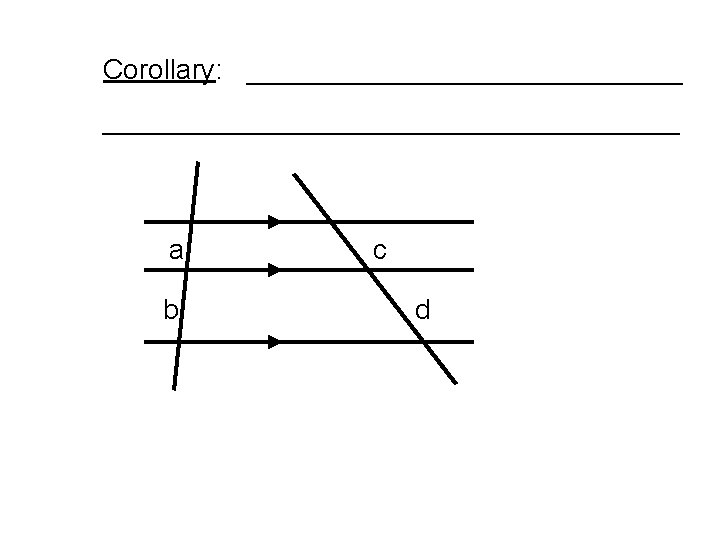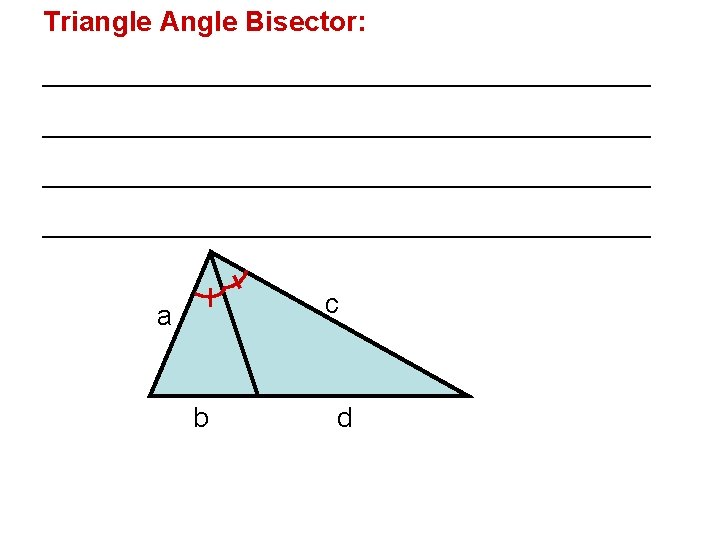Triangle Angle Bisector: _______________________________________ c a b d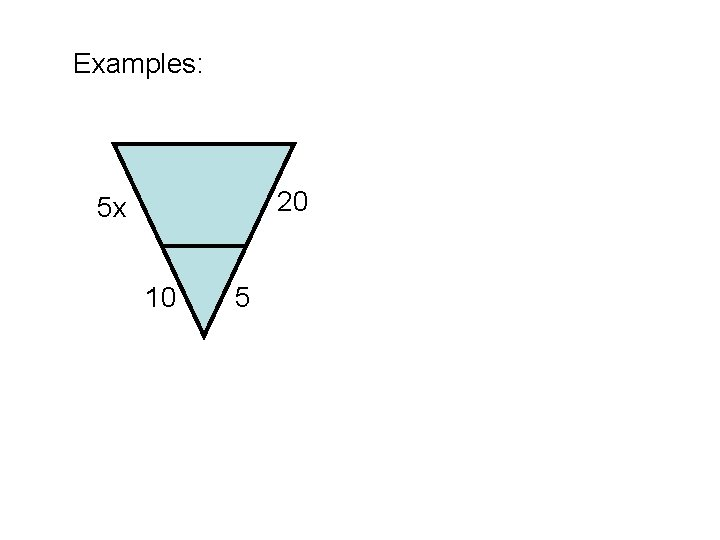Examples: 20 5 x 10 5x 10 12 24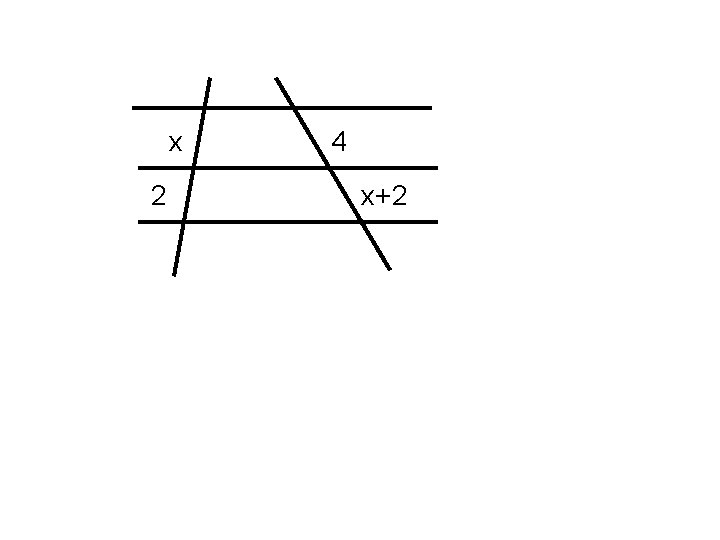x 2 4 x+2